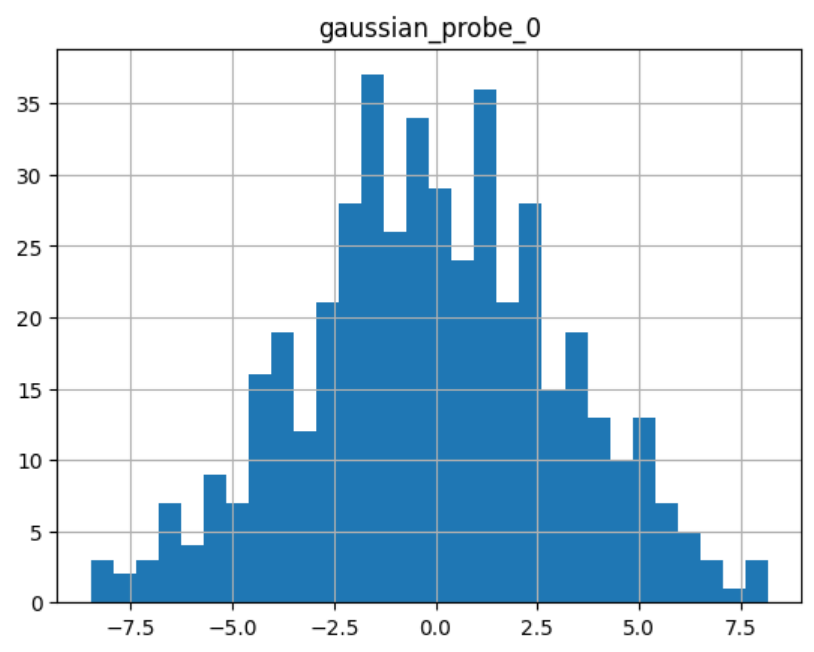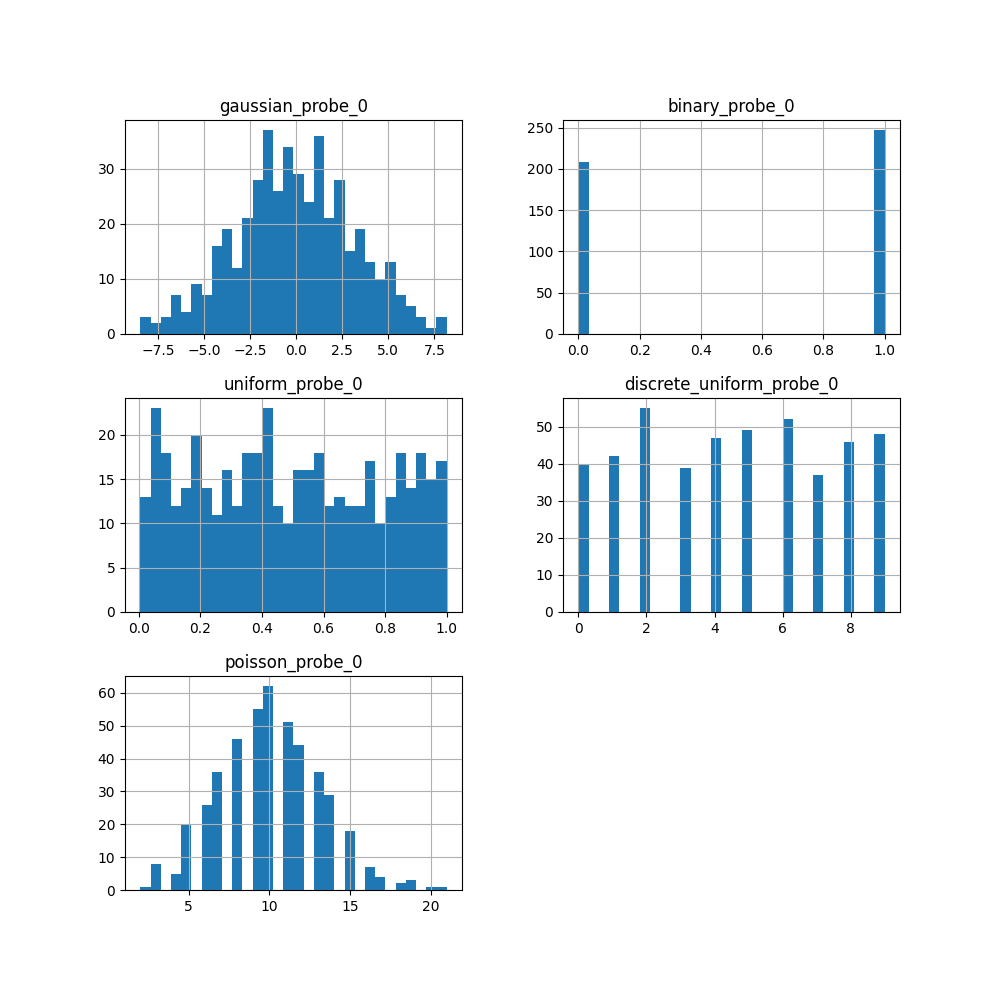# ProbeFeatureSelection#

`ProbeFeatureSelection()` generates one or more random variables based on the user-selected parameters. Next, the transformer derives the feature importance for each variable and probe feature. Finally, it eliminates the features that have a lower feature importance score than the probe feature(s).

In the case of there being more than one probe feature, the average feature importance score of all the probe features is used.

In summary, this is how `ProbeFeatureSelection()` selects features:

1. Create 1 or more random features

2. Train a machine learning model with all features including the random ones

3. Derive feature importance for all features

4. Take the average importance of the random features (only if more than 1 random feature were used)

5. Select features whose importance is greater than the importance of the random variables (step 4)

One of the primary goals of feature selection is to remove noise from the dataset. A randomly generated variable, i.e., probe feature, inherently possesses a high level of noise. Consequently, any variable that demonstrates less importance than a probe feature is assumed to be noise and can be discarded from the dataset.

When initiating the `ProbeFeatureSelection()` class, the user has the option of selecting which distribution is to be assumed to create the probe feature(s) and the number of probe features to be created. The possible distributions are ‘normal’, ‘binary’, ‘uniform’, or ‘all’. ‘all’ creates 1 or more probe features comprised of each distribution type, i.e., normal, binomial, and uniform.

## Example#

Let’s see how to use this transformer to select variables from UC Irvine’s Breast Cancer Wisconsin (Diagnostic) dataset, which can be found here. We will use Scikit-learn to load the dataset. This dataset concerns breast cancer diagnoses. The target variable is binary, i.e., malignant or benign.

The data is solely comprised of numerical data.

Let’s import the required libraries and classes:

```import pandas as pd
from sklearn.ensemble import RandomForestClassifier
from sklearn.model_selection import train_test_split
from feature_engine.selection import ProbeFeatureSelection
```

Let’s now load the cancer diagnostic data:

```cancer_X, cancer_y = load_breast_cancer(return_X_y=True, as_frame=True)
```

Let’s check the shape of `cancer_X`:

```print(cancer_X.shape)
```

We see that the dataset is comprised of 569 observations and 30 features:

```(569, 30)
```

Let’s now split the data into train and test sets:

```# separate train and test sets
X_train, X_test, y_train, y_test = train_test_split(
cancer_X,
cancer_y,
test_size=0.2,
random_state=3
)

X_train.shape, X_test.shape
```

We see the size of the datasets below. Note that there are 30 features in both the training and test sets.

```((455, 30), (114, 30))
```

Now, we set up `ProbeFeatureSelection()`.

We will pass `RandomForestClassifier()` as the `estimator`. We will use `precision` as the `scoring` parameter and `5` as `cv` parameter, both parameters to be used in the cross validation.

In this example, we will introduce just 1 random feature with a normal distribution. Thus, we pass `1` for the `n_probes` parameter and `normal` as the `distribution`.

```sel = ProbeFeatureSelection(
estimator=RandomForestClassifier(),
variables=None,
scoring="precision",
n_probes=1,
distribution="normal",
cv=5,
random_state=150,
confirm_variables=False
)

sel.fit(X_train, y_train)
```

With `fit()`, the transformer:

• creates `n_probes` number of probe features using provided distribution(s)

• uses cross-validation to fit the provided estimator

• calculates the feature importance score for each variable, including probe features

• if there are multiple probe features, the transformer calculates the average importance score

• identifies features to drop because their importance scores are less than that of the probe feature(s)

In the attribute `probe_features`, we find the pseudo-randomly generated variable(s):

```sel.probe_features_.head()
```
```       gaussian_probe_0
0         -0.694150
1          1.171840
2          1.074892
3          1.698733
4          0.498702
```

We can go ahead and display a histogram of the probe feature:

```sel.probe_features_.hist(bins=30)
```

As we can see, it shows a normal distribution:The attribute `feature_importances_` shows each variable’s feature importance:

```sel.feature_importances_.head()
```

These are the first 5 features:

```mean radius        0.058463
mean texture       0.011953
mean perimeter     0.069516
mean area          0.050947
mean smoothness    0.004974
```

At the end of the series, we see the importance of the probe feature:

```sel.feature_importances_.tail()
```

These are the importance of the last 5 features including the probe:

```worst concavity            0.037844
worst concave points       0.102769
worst symmetry             0.011587
worst fractal dimension    0.007456
gaussian_probe_0           0.003783
dtype: float64
```

In the attribute `features_to_drop_`, we find the variables that were not selected:

```sel.features_to_drop_
```

These are the variables that will be removed from the dataframe:

```['mean symmetry',
'mean fractal dimension',
'texture error',
'smoothness error',
'concave points error',
'fractal dimension error']
```

We see that the `features_to_drop_` have feature importance scores that are less than the probe feature’s score:

```sel.feature_importances_.loc[sel.features_to_drop_+["gaussian_probe_0"]]
```

The previous command returns the following output:

```mean symmetry              0.003698
mean fractal dimension     0.003455
texture error              0.003595
smoothness error           0.003333
concave points error       0.003548
fractal dimension error    0.003576
gaussian_probe_0           0.003783
```

With `transform()`, we can go ahead and drop the six features with feature importance score less than `gaussian_probe_0` variable:

```Xtr = sel.transform(X_test)

Xtr.shape
```

The final shape of the data after removing the features:

```(114, 24)
```

And, finally, we can also obtain the names of the features in the final transformed dataset:

```sel.get_feature_names_out()

'mean texture',
'mean perimeter',
'mean area',
'mean smoothness',
'mean compactness',
'mean concavity',
'mean concave points',
'perimeter error',
'area error',
'compactness error',
'concavity error',
'symmetry error',
'worst texture',
'worst perimeter',
'worst area',
'worst smoothness',
'worst compactness',
'worst concavity',
'worst concave points',
'worst symmetry',
'worst fractal dimension']
```

For compatibility with Scikit-learn selection transformers, `ProbeFeatureSelection()` also supports the method `get_support()`:

```sel.get_support()
```

which returns the following output:

```[True, True, True, True, True, True, True, True, False, False, True, False, True,
True, False, True, True, False, True, False, True, True, True, True, True, True,
True, True, True, True]
```

### Using several probe features#

Let’s now repeat the selection process, but using more than 1 probe feature.

```sel = ProbeFeatureSelection(
estimator=RandomForestClassifier(),
variables=None,
scoring="precision",
n_probes=3,
distribution="all",
cv=5,
random_state=150,
confirm_variables=False
)

sel.fit(X_train, y_train)
```

Let’s display the random features that the transformer created:

```sel.probe_features_.head()
```

Here we find some example values of the probe features:

```   gaussian_probe_0  binary_probe_0  uniform_probe_0
0         -0.694150               1         0.983610
1          1.171840               1         0.765628
2          1.074892               1         0.991439
3          1.698733               0         0.668574
4          0.498702               0         0.192840
```

Let’s go ahead and plot histograms:

```sel.probe_features_.hist(bins=30)
```

In the histograms we recognise the 3 well defined distributions:Let’s display the importance of the random features

```sel.feature_importances_.tail()
```
```worst symmetry             0.009176
worst fractal dimension    0.007825
gaussian_probe_0           0.003765
binary_probe_0             0.000354
uniform_probe_0            0.002377
dtype: float64
```

We see that the binary feature has an extremely low importance, hence, when we take the average, the value is so small, that no feature will be dropped:

```sel.features_to_drop_
```

The previous command returns and empty list:

```[]
```

It is important to select a suitable probe feature distribution when trying to remove variables. If most variables are continuous, introduce features with normal and uniform distributions. If you have one hot encoded features or sparse matrices, binary features might be a better option.